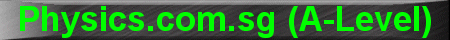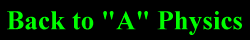(New tips are continually added to these pages.  Check back in a few months' time for more)

TOPIC 5:    Temperature, Thermodynamics and Ideal Gases

Tip 1:

Some students, especially those who are very hardworking and read multiple text books, are sometimes confused as to why there are 2 forms of the thermodynamic equation (First Law of Thermodynamics).

The 2 forms are:

DU = Q + W

Q = DU + W

The first equation is the one that is commonly taught in JCs.

In the first equation, W represents the work done ON the gas - it is positive if the gas is compressed but negative if the gas expands; whereas in the second equation, W represents the work done BY the gas - it is positive if the gas expands but negative if the gas is compressed.

Tip 2:

Sometimes, examiners like to combine different chapters to make problems more challenging.

For example, they may set a thermodynamics question without giving the value for change in internal energy DU, but with data on temperature and number of moles of gas.

In this case, we can use one of the equations from the chapter on Ideal Gases:

DU = 3/2 n R DT

to calculate the change in internal energy DU, provided that the system is an ideal gas (ie. only K.E. but no P.E.)